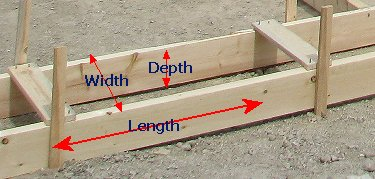Spike's Calculators

# Metric Concrete Calculator for Footings

Calculate the amount of concrete needed for pouring footings using metric measurements.##### Formula's used:
`length * width * depth = Volume`

##### Example calculation:
```footings length = 200 m
width = 600 mm
footings depth = 100 mm
```

To be able to do a straight calculation, the units you use have to be the same and when you are using metric units, that is a simple step.

Change the millimetres to metres by dividing by 1000 (one metre = 1000 millimetres)
```600 ÷ 1000 = 0.600 metres
100 ÷ 1000 = 0.100 metres```
Now using the above formula, you can easily calculate the volume of the footings in cubic meters;
`200 * 0.6 * 0.1 = 12`
You need 12 cubic metres of concrete.

To convert cubic metres to cubic yards multiply cubic metres * 1.30795061931439
To convert cubic metres to cubic feet multiply cubic metres * 35.3146667214886

### Concrete Footings

Length of Footings m
Width mm
Depth of Footings mm

 Perimeter of Footings m Amount of Concrete Needed in: Cubic Metres m³ Cubic Yards yd ³ Cubic Feet ft ³

#### Calculation

1. enter the length of the footings in metres
2. the width of the footings in millimetres
3. depth of footings in millimetres

#### Results

1. perimeter of the footings (for the forming material needed in lineal metres)
2. amount of concrete needed in cubic metres
3. concrete needed in cubic yards
4. concrete needed in cubic feet

### When Ordering Concrete

This concrete calculator will help you in estimating the amount of concrete needed for your project. The amount given as needed, does not include any waste. It is recommended, depending on the job you are doing, to add anywhere from an extra 4% to 10% to your concrete order to make sure you have enough concrete to finish the job. Ask when ordering the concrete. They will be able to give you a good idea of what is needed.